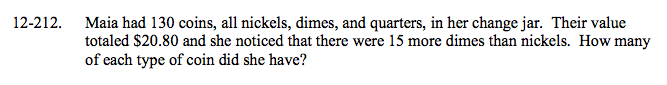### Home > A2C > Chapter 12 > Lesson 12.5.2 > Problem12-212

12-212.

Maia had 130 coins, all nickels, dimes, and quarters, in her change jar. Their value totaled \$20.80 and she noticed that there were 15 more dimes than nickels. How many of each type of coin did she have? Homework Help ✎Write 3 equations to describe the situation.

n + d + q = 130
0.05n + 0.10d + 0.25q = 20.80
n + 15 = d